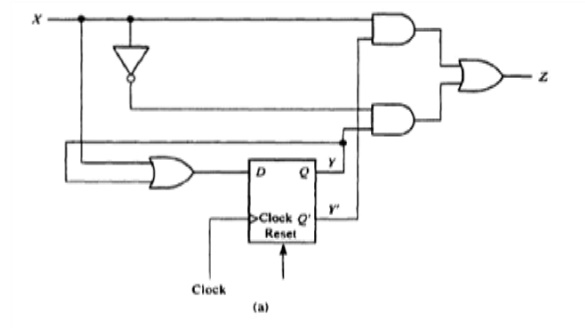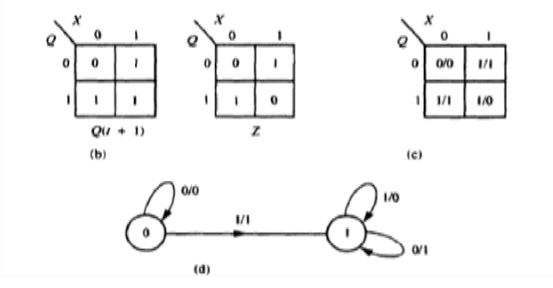Home | | Digital Logic Circuits | Analysis and Synthesis of Synchronous Sequential Circuit

# Analysis and Synthesis of Synchronous Sequential Circuit

The analysis of a synchronous sequential circuit is the process of determining the functional relation that exists between its outputs, and its internal states.

Analysis and Synthesis of Synchronous Sequential Circuit

The analysis of a synchronous sequential circuit is the process of determining the functional relation that exists between its outputs, and its internal states. The contents of all the flip flops in the circuit combined determine the internal state of the circuit. Thus, if the circuit contains n flip flops. It can be in one of the 2n states. Knowing the present state of the circuit and the input values at any time t, We should be able to derive its next state (i.e.. the state at time t+1) and the output produced by the circuit at t.

A sequential circuit can be described completely by a state table that is very similar to the ones shown for flip flops

For a circuit can be 2n rows in the state table. If there are m inputs to the circuit, there will be 2n column in the state table. At the intersection of each row and column, the next stable and the output information are recorded. A state diagram is a graphical representation of the state table in which each state is represented by a circle and the state transitions are represented by arrows between the circles. The input combination that brings about the transition and the corresponding output information are shown on the arrow. Analysing a sequential circuit thus corresponds to generating the state table and the state diagram for the circuit. The state table or state diagram can be used to determine to output sequence generated by the circuit for a given input sequence if the initial state it known. It is important to note that for proper operation, a sequential circuit must be in its initial state before the inputs to it can be applied. Usually the power up circuits are used to initialize the circuit to the appropriate state when the never is turned on.Sequential circuit analysis (a) circuit (b) next state and output tables (c) transition table (d) state diagram (e) timing diagram for level input (f) timing diagram for synchronous pulse input.

Study Material, Lecturing Notes, Assignment, Reference, Wiki description explanation, brief detail
Digital Logic Circuits : Synchronous Sequential Circuits : Analysis and Synthesis of Synchronous Sequential Circuit |